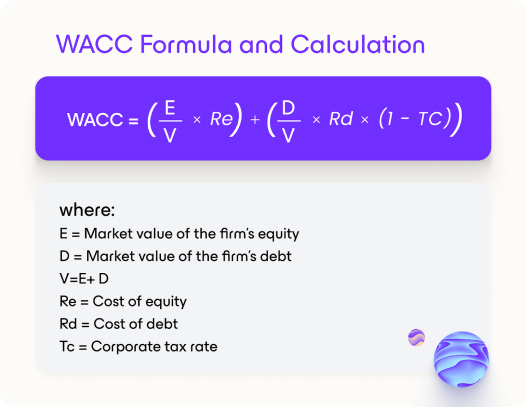# How to calculate the weighted average cost of capital (WACC)Afshan Qureshi
Content Marketing Manager
Posted on
May 27, 2022
·
5## What Is the weighted average cost of capital (WACC)

Raising capital comes at a cost; to better understand this cost, we use the weighted average cost of capital (WACC) to define the average post-tax cost of a company’s two main sources of capital: debt and equity (including, but not limited to common stock, preferred stock, bonds, and any other long-term debt).

This metric is a common and insightful way to calculate the required rate of return because it culminates the return that’s require to be paid in into a single number to use as a benchmark. Ultimately, WACC is the average rate a business anticipates paying to finance its assets since the cost associated with each type of capital can vary greatly.

## What we learn from the weighted average cost of capital (WACC)

Finance departments calculate a company’s cost of capital for a few vital reasons.

Firstly, WACC is the discount rate that a company uses to estimate its net value at present. The weighted average cost of capital calculator formula is used by founders and investors to determine an investor's returns on an investment in a company.

Businesses usually run on borrowed funds, so the cost of capital calculator becomes an important tool in determining a company’s potential for net profitability. WACC measures a company’s cost to borrow money, whereas the WACC formula uses both the company’s debt and equity in its calculation. Essentially, the WACC formula provides insight into how much interest a company owes for each dollar it borrows.Maintain independence and raise money your way

WACC is also a key metric used in discounted cash flow (DCF) analysis. The WACC metric can be used to determine which projects a company should undertake. If the company believes that a merger might generate a return higher than its cost of capital, it’s likely a good choice for the company to sell and merge at that time.

If it’s determined that the return will be lower than what their own investors are expecting, then they’ll want to put their capital to better use elsewhere.

Lastly, investors use WACC to assess if an investment is viable or not. The lower the WACC the better, since it signals a healthy business that’s ready to attract investment at a lower cost.

On the other hand, a higher WACC usually means that a business is riskier and will need to compensate investors with higher returns in order to attract funding. The WACC will be higher if a company’s stock is volatile or if its debt is riskier.

## How to calculate the weighted average cost of capital (WACC)

The WACC formula consists of the weighted average cost of equity plus the weighted average cost of debt. Usually, the cost of debt is lower than the cost of equity because interest expenses can be tax-deductible.

Calculating WACC usually uses the market value of the various components in the formula versus the book values because the expected cost of new capital is of higher importance than the sale of existing assets. It’s important to remember that the lower the WACC, the better.Example: a company obtained \$1,000,000 in debt financing and \$4,000,000 in equity financing by selling common shares. E/V would equal 0.8 (\$4,000,000 ÷ \$5,000,000 of total capital) and D/V would equal 0.2 (\$1,000,000 ÷ \$5,000,000 of total capital).

## Limitations of the weighted average cost of capital (WACC)

The WACC can be hard to calculate, especially if you're not familiar with all the inputs. Higher debt levels usually mean a higher WACC will be required. Complex balance sheets with varying types of debt and interest rates will find it more complicated to calculate their WACC.

Additionally, the inputs for the WACC calculation can all be affected by market and economic conditions, which adds further nuance to the calculation and metric.

A downside to the WACC is the assumption that the current capital structure doesn’t change. Another limitation of WACC is that there is more than one way to calculate it, which adds further complication to the metric.

## Final thoughts on the weighted average cost of capital (WACC)

The WACC formula seems easier to calculate than it is in reality. This is because some parts of the formula, like the cost of equity, aren’t always consistent. Even though WACC provides valuable insight into a company, it should be used in tandem with other metrics when analyzing if a company should be invested in.

Ultimately, WACC is a formula that’s useful to analysts, investors, and founders, all of whom use it differently.